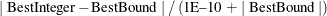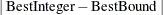# The OPTMILP Procedure

### Macro Variable _OROPTMILP_

The OPTMILP procedure defines a macro variable named _OROPTMILP_. This variable contains a character string that indicates the status of the OPTMILP procedure upon termination. The various terms of the variable are interpreted as follows.

STATUS

indicates the solver status at termination. It can take one of the following values:

OK

The procedure terminated normally.

SYNTAX_ERROR

Incorrect syntax was used.

DATA_ERROR

The input data was inconsistent.

OUT_OF_MEMORY

Insufficient memory was allocated to the procedure.

IO_ERROR

A problem occurred in reading or writing data.

ERROR

The status cannot be classified into any of the preceding categories.

ALGORITHM

indicates the algorithm that produced the solution data in the macro variable. This term only appears when STATUS=OK. It can take one of the following values:

BAC

The branch-and-cut algorithm produced the solution data.

DECOMP

The decomposition algorithm produced the solution data.

SOLUTION_STATUS

indicates the solution status at termination. It can take one of the following values:

OPTIMAL

The solution is optimal.

OPTIMAL_AGAP

The solution is optimal within the absolute gap specified by the ABSOBJGAP= option.

OPTIMAL_RGAP

The solution is optimal within the relative gap specified by the RELOBJGAP= option.

OPTIMAL_COND

The solution is optimal, but some infeasibilities (primal, bound, or integer) exceed tolerances due to scaling or choice of a small INTTOL= value.

TARGET

The solution is not worse than the target specified by the TARGET= option.

INFEASIBLE

The problem is infeasible.

UNBOUNDED

The problem is unbounded.

INFEASIBLE_OR_UNBOUNDED

The problem is infeasible or unbounded.

SOLUTION_LIM

The solver reached the maximum number of solutions specified by the MAXSOLS= option.

NODE_LIM_SOL

The solver reached the maximum number of nodes specified by the MAXNODES= option and found a solution.

NODE_LIM_NOSOL

The solver reached the maximum number of nodes specified by the MAXNODES= option and did not find a solution.

TIME_LIM_SOL

The solver reached the execution time limit specified by the MAXTIME= option and found a solution.

TIME_LIM_NOSOL

The solver reached the execution time limit specified by the MAXTIME= option and did not find a solution.

ABORT_SOL

The solver was stopped by the user but still found a solution.

ABORT_NOSOL

The solver was stopped by the user and did not find a solution.

OUTMEM_SOL

The solver ran out of memory but still found a solution.

OUTMEM_NOSOL

The solver ran out of memory and either did not find a solution or failed to output the solution due to insufficient memory.

FAIL_SOL

The solver stopped due to errors but still found a solution.

FAIL_NOSOL

The solver stopped due to errors and did not find a solution.

OBJECTIVE

indicates the objective value obtained by the solver at termination.

RELATIVE_GAP

specifies the relative gap between the best integer objective (BestInteger) and the objective of the best remaining node (BestBound) upon termination of the OPTMILP procedure. The relative gap is equal toABSOLUTE_GAP

specifies the absolute gap between the best integer objective (BestInteger) and the objective of the best remaining node (BestBound) upon termination of the OPTMILP procedure. The absolute gap is equal to.

PRIMAL_INFEASIBILITY

indicates the maximum (absolute) violation of the primal constraints by the solution.

BOUND_INFEASIBILITY

indicates the maximum (absolute) violation by the solution of the lower or upper bounds (or both).

INTEGER_INFEASIBILITY

indicates the maximum (absolute) violation of the integrality of integer variables returned by the OPTMILP procedure.

BEST_BOUND

specifies the best LP objective value of all unprocessed nodes on the branch-and-bound tree at the end of execution. A missing value indicates that the OPTMILP procedure has processed either all or none of the nodes on the branch-and-bound tree.

NODES

specifies the number of nodes enumerated by the OPTMILP procedure when using the branch-and-bound algorithm.

ITERATIONS

indicates the number of simplex iterations taken to solve the problem.

PRESOLVE_TIME

indicates the time (in seconds) used in preprocessing.

SOLUTION_TIME

indicates the time (in seconds) taken to solve the problem, including preprocessing time.

Note: The time reported in PRESOLVE_TIME and SOLUTION_TIME is either CPU time or real time. The type is determined by the TIMETYPE= option.